﻿ 大口径望远镜消旋K镜旋转策略及准直技术研究 Rotating Strategy and Alignment Technology of K Mirror in Large Aperture Telescope

Optoelectronics
Vol. 09  No. 03 ( 2019 ), Article ID: 32299 , 12 pages
10.12677/OE.2019.93019

Rotating Strategy and Alignment Technology of K Mirror in Large Aperture Telescope

Yan Zhang, Baogang Chen, Hongwen Li, Liang Wang

Changchun Institute of Optics, Fine Mechanics and Physics, Chinese Academy of Sciences, Changchun Jilin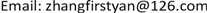Received: Sep. 2nd, 2019; accepted: Sep. 17th, 2019; published: Sep. 24th, 2019ABSTRACT

Aiming at the image field rotation of 2 m Alt-az telescope, the K mirror is designed. First, according to optical transfer characteristic of K mirror system, the mathematical matrix model is calculated. The second, based on the method of ray tracing, the transformation relation between incident light coordinate and coude focus coordinate is fitted. The image field rotation of incident light through the light path of coude is analyzed, and the conversion regularity of the image field rotation involving the azimuth axis and pitching axis of telescope is deduced, thus the initial zero and rotation rules of K mirror are obtained. Then, in allusion to the alignment accuracy of K mirror, through the analysis of the periodic variation characteristics of alignment error in the rotation period of K mirror, the alignment adjustment strategy of K mirror is designed basing on the theory of Pascal Spiral, the complex problem of alignment adjustable is transformed into the parameter correction of mathematical equations, and the calculation model of the alignment accuracy and the key parameters in Pascal Spiral equation is established. Finally, the alignment adjustable experiment is carried out. The key parameters in the model are modified repeatedly during the experiment. The alignment error curve keeps getting closer and closer to the optimal value. The correctness and effectiveness of the research is verified from the experimental results.

Keywords:Alt-az Telescope, K Mirror, Coude Path, Alignment, Pascal Spiral1. 引言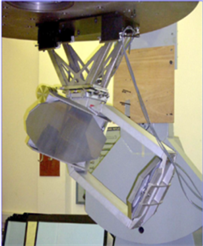(a) K mirror in JCMT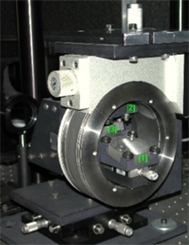(b) k mirror in Hooker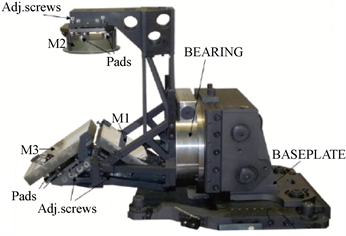(c) k mirror in LBT

Figure 1. The K mirror in large aperture telescope

2. K镜光学消旋数学模型

${R}_{k}=I-2{N}_{k}\cdot {N}_{k}^{\text{T}}$ (1)

$\begin{array}{l}{N}_{k1}=\left[-\mathrm{cos}\alpha ,0,-\mathrm{sin}\alpha \right]\\ {N}_{k2}=\left[\mathrm{cos}\beta ,0,\mathrm{sin}\beta \right]\\ {N}_{k3}=\left[-\mathrm{cos}\gamma ,0,\mathrm{sin}\gamma \right]\end{array}$ (2)

${A}^{\prime }={R}_{k3}{R}_{k2}{R}_{k1}A$ (3)

$A=\left[\begin{array}{ccc}\mathrm{cos}\theta & -\mathrm{sin}\theta & 0\\ \mathrm{sin}\theta & \mathrm{cos}\theta & 0\\ 0& 0& 1\end{array}\right]\left[\begin{array}{c}{A}_{x}\\ {A}_{y}\\ {A}_{z}\end{array}\right]$ (4)

K镜中，K1、K2、K3反射镜作用矩阵则相应地变为：

$\begin{array}{l}{N}_{k1}=\left[\begin{array}{ccc}\mathrm{cos}\theta /2& -\mathrm{sin}\theta /2& 0\\ \mathrm{sin}\theta /2& \mathrm{cos}\theta /2& 0\\ 0& 0& 1\end{array}\right]\left[\begin{array}{c}-\mathrm{cos}\alpha \\ 0\\ -\mathrm{sin}\alpha \end{array}\right]\\ {N}_{k2}=\left[\begin{array}{ccc}\mathrm{cos}\theta /2& -\mathrm{sin}\theta /2& 0\\ \mathrm{sin}\theta /2& \mathrm{cos}\theta /2& 0\\ 0& 0& 1\end{array}\right]\left[\begin{array}{c}\mathrm{cos}\beta \\ 0\\ \mathrm{sin}\beta \end{array}\right]\\ {N}_{k3}=\left[\begin{array}{ccc}\mathrm{cos}\theta /2& -\mathrm{sin}\theta /2& 0\\ \mathrm{sin}\theta /2& \mathrm{cos}\theta /2& 0\\ 0& 0& 1\end{array}\right]\left[\begin{array}{c}-\mathrm{cos}\gamma \\ 0\\ \mathrm{sin}\gamma \end{array}\right]\end{array}$ (5)

K镜系统内部光学元件的位置关系一般满足一下条件：

$\alpha =\gamma ,\beta =0$ (6)

${A}^{\prime }={R}_{k3}{R}_{k2}{R}_{k1}A=\left[\begin{array}{ccc}-1& 0& 0\\ 0& 1& 0\\ 0& 0& 1\end{array}\right]\left[\begin{array}{c}{A}_{x}\\ {A}_{y}\\ {A}_{z}\end{array}\right]$ (7)Figure 2. Light path of K mirror

${R}_{k3}{R}_{k2}{R}_{k1}=\left[\begin{array}{ccc}-1& 0& 0\\ 0& 1& 0\\ 0& 0& 1\end{array}\right]$ (8)

$\left[\begin{array}{c}1-2{\mathrm{cos}}^{2}\gamma \\ 1\\ 1-2{\mathrm{sin}}^{2}\gamma \end{array}\right]\left[\begin{array}{c}1-2{\mathrm{cos}}^{2}\beta \\ 1\\ 1-2{\mathrm{sin}}^{2}\beta \end{array}\right]\left[\begin{array}{c}1-2{\mathrm{cos}}^{2}\alpha \\ 1\\ 1-2{\mathrm{sin}}^{2}\alpha \end{array}\right]=\left[\begin{array}{ccc}-1& 0& 0\\ 0& 1& 0\\ 0& 0& 1\end{array}\right]$ (9)

$±\left(\alpha -\gamma \right)=\beta$ (10)

3. K镜消旋策略研究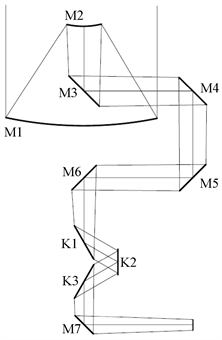Figure 3. Light path of 2m telescope

2 m望远镜采用地平坐标，A为方位角，从正北向东计量，范围0˚~360˚，对应于方位轴的转动。Z为天顶距，从天顶向前下方计量，范围为0˚~90˚，对应于俯仰轴的转动。定义望远镜主光路入瞳处坐标系为o-xyz，坐标系中角度的方向，迎着光线(逆光)观察逆时针方向为正方向。从坐标系o-xyz中分别发出四条光线x+、x−、y+、y−，沿主光路方向最终汇聚至coude像面，光线坐标系o-xyz经过M3、M4、M5、M6、M7镜之后分别形成局部坐标系o3-x3y3z3、o4-x4y4z4、o5-x5y5z5、o6-x6y6z6、o7-x7y7z7。如图4所示。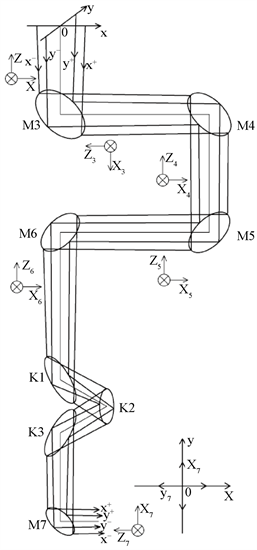Figure 4. COUDE path of 2 m telescope

M3镜固定在2 m主镜之上，其随望远镜方位轴及俯仰轴转动，M4、M5、M6镜安装在望远镜水平轴立柱上，其随望远镜方位轴转动，当望远镜俯仰轴旋转时，其保持固定状态。K镜放置于M6镜之后，因此我们要建立主光路经过M6镜之后的像面旋转与望远镜俯仰轴、方位轴旋转的关系。

$\Delta \theta =\Delta A-\Delta Z$ (11)

$\omega =\frac{\Delta \theta }{\Delta t}=\frac{\Delta A-\Delta Z}{\Delta t}={\omega }_{A}-{\omega }_{Z}$ (12)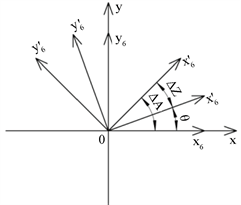Figure 5. Rotation schematic diagram of local coordinate system

${\omega }_{K}=1/2\frac{\Delta \theta }{\Delta t}=1/2\left({\omega }_{A}-{\omega }_{Z}\right)$ (13)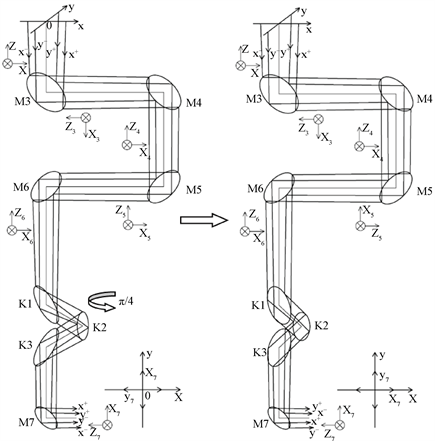Figure 6. The ray tracing of coude path

4. 准直技术及误差分析

2 m望远镜在跟踪目标过程中，由于俯仰、方位轴的转动，光线在2 m库德光路中会相应的发生旋转，导致望远镜成像焦点处产生像旋。为避免库德焦点处的图像旋转，需要在2 m主光路中引入K镜系统。为保证K镜具有最好的消旋效果，必须要确保K镜光轴、K镜机械回转轴及2 m望远镜主光轴三轴重合，本节对K镜的准直技术及误差分析展开深入研究。

$\Delta {D}_{3}=2\left(\Delta {D}_{1}\mathrm{cos}\theta -\Delta {D}_{2}\right)$ (14)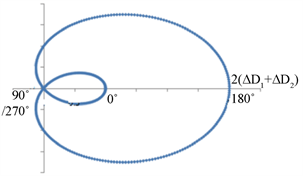(a) ∆D1≠0,∆D2≠0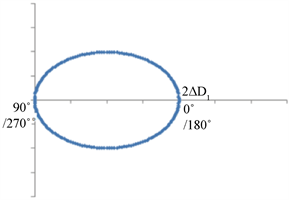(b) ∆D1 ≠0,∆D2 =0

Figure 7. The alignment error curve of K mirror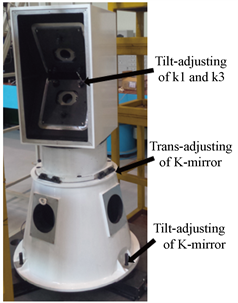Figure 8. The mechanical structure of K mirror

2 m望远镜K镜系统放置于M6镜与M7镜之间。在主光路中放置具有自调焦功能的测微准直望远镜(CZW)，在K镜之后沿主光路分别放置2个CCD，用于记录主光路经过K镜后的位移轨迹，如图9图10所示。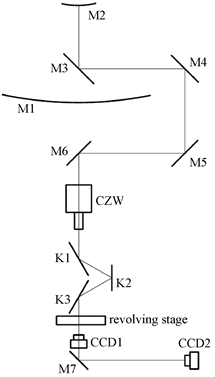Figure 9. The sketch of alignment adjustment strategy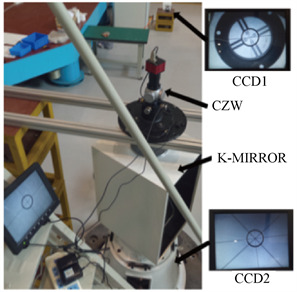Figure 10. Alignment adjustable experiment of K mirror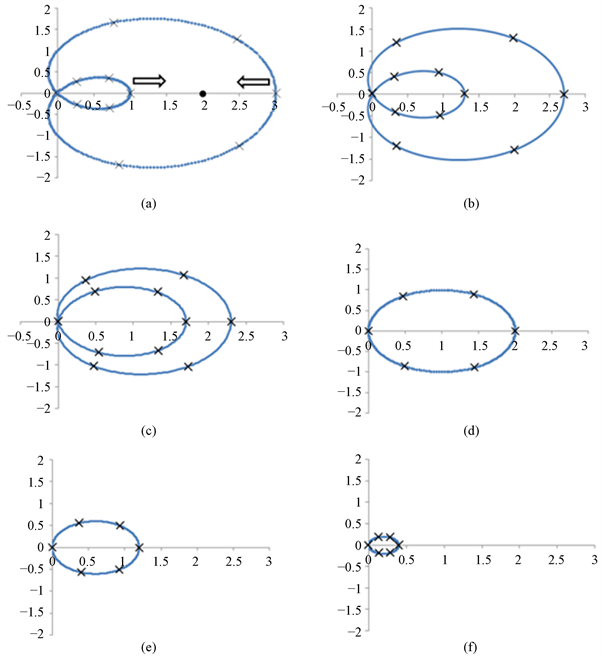Figure 11. Light-spot recording in the process of alignment calibration (CCD2)

$\sigma ={\mathrm{tan}}^{-1}\left(\frac{\Delta {D}_{3}}{H}\right)=4″$ (14)

5. 结论

Rotating Strategy and Alignment Technology of K Mirror in Large Aperture Telescope[J]. 光电子, 2019, 09(03): 129-140. https://doi.org/10.12677/OE.2019.93019

1. 1. 张景旭. 地基大口径望远镜系统结构技术综述[J]. 中国光学, 2012, 5(4): 327-336.

2. 2. 赵勇志, 邵亮, 明名, 等. 大口径望远镜主镜支撑系统装调[J]. 红外与激光工程, 2017, 46(9): 267-274.

3. 3. 安其昌, 张景旭, 杨飞, 等. 基于结构函数的大口径望远镜中频误差分配研究[J]. 光学精密工程, 2017, 25(2): 433-440.

4. 4. Brunelli, A., Bergomi, M., Dima, M., et al. (2012) Tips & Tricks for Aligning an Image Derotator. Proceedings of SPIE, 8446, 8446L1-8446L10. https://doi.org/10.1117/12.926884

5. 5. 惠刚阳, 杨海成, 姜峰, 等. 潜望式瞄准镜中消像旋棱镜的装调工艺技术[J]. 应用光学, 2013, 34(4): 700-705.

6. 6. Altmann, B., Pape, C. and Reithmeier, E. (2017) Temperature Measurements on Fast-Rotating Objects Using a Thermographic Camera with an Optomechanical Image Derotator. Proceedings of SPIE, 10404, 1-7. https://doi.org/10.1117/12.2271817

7. 7. 王岱, 李晓燕, 吴钦章. 某光测设备上消像旋的设计[J]. 光电工程, 2012, 39(1): 108-112.

8. 8. 鞠青华, 李语强, 熊耀恒. 1.2m地平式望远镜视场旋转角的理论计算[J]. 天文研究与技术, 2009, 6(1): 28-35.

9. 9. 魏烨艳, 王东光, 梁明, 等. 天文望远镜消旋K镜光学结构设计及分析[J]. 中国激光, 2016, 43(3): 250-259.

10. 10. 郭鹏, 张景旭, 杨飞, 等. 2m望远镜K镜支撑结构优化设计[J]. 红外与激光工程, 2014, 43(6): 1914-1919.

11. 11. Baudet, J., Jolissaint, L., Keskin, O., et al. (2017) Design of a Derotator for 4m DAG Telescope. Proceedings of SPIE, 9908, 1-6. https://doi.org/10.1117/12.2234392

12. 12. Barboza, S., Pott, J.-U., Rohloff, R.-R., et al. (2016) Design of a Large Image Derotator for the E-ELT Instrument MICADO. Proceedings of SPIE, 9908, 1-14. https://doi.org/10.1117/12.2231632

13. 13. Xu, J., Ren, C. and Ye, Y. (2017) Optics Derotator Servo Control System for SONG Telescope. Proceedings of SPIE, 8444, 1-7.

14. 14. Paul, R., Yoder, Jr. 光机系统设计[M]. 北京: 机械工程出版社, 2013.